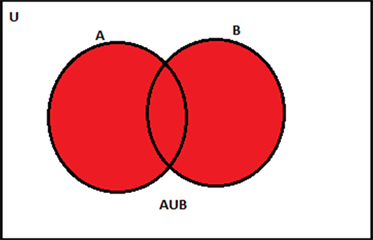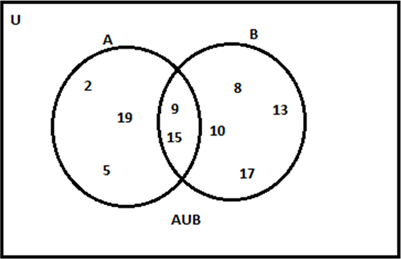Checkout JEE MAINS 2022 Question Paper Analysis : Checkout JEE MAINS 2022 Question Paper Analysis :

# Union Of Sets

Union of two or more sets is the set containing all the elements of the given sets. Union of sets can be written using the symbol “⋃”. Suppose the union of two sets X and Y can be represented as X ⋃ Y. As we know, sets can undergo different operations and the basic operations that can be performed on sets are as follows:

1. Union of sets
2. Intersection of sets
3. Difference of sets

In mathematics, we perform certain operations like addition, subtraction, multiplication, etc. These operators generally take two or more operands and give a result based on the operation performed. Similarly, in set theory usually, certain operations are performed on two or more sets to get a new set of elements based on the operation performed. The union and intersection of sets represent the number of elements carried by the operation and executing the result of a collective set. In the case of union, all the elements are included in the result but in the case of the intersection, only the common elements are considered.

Sometimes, students also get confused with the union and universal set. Union of two or more sets is an operation performed on them, which results in a collection of elements present in both the sets, whereas a universal set is itself a set, which contains all the elements of other sets, including its own elements.

In the upcoming discussions, you will learn about the union operation on sets thoroughly.

## Union of Sets Definition

The union of two sets X and Y is equal to the set of elements that are present in set X, in set Y, or in both the sets X and Y. This operation can be represented as;

X ∪ Y = {a: a ∈ X or a ∈ Y}

Let us consider an example, say;  set A = {1, 3, 5} and set B = {1, 2, 4} then;

A ∪ B = {1, 2, 3, 4, 5}

Now, let us learn how can we represent the union of two sets in a Venn diagram.

### Venn Diagram of Union of Sets

Let us consider a universal set U such that A and B are the subsets of this universal set. The union of two sets A and B is defined as the set of all the elements which lie in set A and set B or both the elements in A and B altogether. The union of the set is denoted by the symbol ‘∪’.In the given Venn diagram, the red-coloured portion represents the union of both sets A and B.

Thus, the union of two sets A and B is given by a set C, which is also a subset of the universal set U such that C consists of all those elements or members which are either in set A or set B or in both A and B i.e.,

C = A ∪ B = {x : x ∈ A or x ∈ B}

## A Union B Formula

The union of set A and set B is equal to the set containing all the elements in A and B. This is represented as A U B and can be read as “A union B” or “A or B”.

A union B formula is generally used to calculate the unions of set A and set B. The formula for A union B indicates that each element present in A or B (leaving duplicates) is present in A U B. From the definition of the union of sets, the formula for A union B formula can be written as:

A U B = {x : x ∈ A or x ∈ B}

### Formula for Number of Elements in A union B

Consider two sets, A and B, such that the number of elements in the union of A and B can be calculated as follows.

n(A U B) = n(A) + n(B) – n(A ∩ B)

Here,

n(A U B) = Total number of elements in A U B; is called the cardinality of a set A U B

n(A) = Number of elements in A; is called the cardinality of set A

n(B) = Number of elements in B; is called the cardinality of set B

n(A ∩ B) = The number of elements that are common to both A and B; is called the cardinality of set A ∩ B, i.e. A intersection B

Learn: Intersection of sets

### Properties of Union of  Sets

i)   Commutative Law: The union of two or more sets follows the commutative law i.e., if we have two sets A and B then,

A∪B=B∪A

Example: A = {a, b} and B = {b, c, d}

So, A∪B = {a, b, c, d}

B∪A = {b, c, d, a}

Since, in both the union, the group of elements is same. Therefore, it satisfies commutative law.

A ∪ B = B ∪ A

ii) Associative Law: The union operation follows the associative law i.e., if we have three sets A, B and C then

(A ∪ B) ∪ C = A ∪ (B ∪ C)

Example: A = {a, b} and B = {b, c, d} and C = {a, c, e}

(A ∪ B) ∪ C = {a, b, c, d} ∪ {a, c, e} = {a, b, c, d, e}

A ∪ (B ∪ C) = {a, b} ∪ {b, c, d, e} = {a, b, c, d, e}

Hence, associative law proved.

iii)  Identity Law: The union of an empty set with any set A gives the set itself i.e.,

A ∪ ∅ = A

Suppose, A = {a, b, c} and ∅ = {}

then, A ∪ ∅ = {a, b, c} ∪ {} = {a, b, c}

iv)  Idempotent Law: The union of any set A with itself gives the set A i.e.,

A ∪ A = A

Suppose, A = {1, 2, 3, 4, 5}

then A ∪ A = {1, 2, 3, 4, 5} ∪ {1, 2, 3, 4, 5} = {1, 2, 3, 4, 5} = A

v)  Domination Law: The union of a universal set U with its subset A gives the universal set itself.

A ∪ U = U

Suppose, A = {1, 2, 4, 7} and U = {1, 2, 3, 4, 5, 6, 7}

then A ∪ U = {1, 2, 4, 7} ∪ {1, 2, 3, 4, 5, 6, 7} = {1, 2, 3, 4, 5, 6, 7} = U

Hence, proved.

Go through the solved examples given below to understand the concept in a better way.

### Solved Examples

Example 1:

Let U be a universal set consisting of all the natural numbers until 20 and set A and B be a subset of U defined as A = {2, 5, 9, 15, 19} and B = {8, 9, 10, 13, 15, 17}. Find A ∪ B.

Solution:

Given,

U = {1, 2, 3, 4, 5, 6, 7, 8, 9, 10, 11, 12, 13, 14, 15, 16, 17, 18, 19, 20}

A = {2, 5, 9, 15, 19}

B = {8, 9, 10, 13, 15, 17}

A ∪ B = {2, 5, 8, 9, 10, 13, 15, 17, 19}

This can be represented using the following Venn diagram:Example 2:

If set A contains 13 elements, set B contains 8 elements and the intersection of these two sets contains 5 elements, then find the number of elements in A union B.

Solution:

Given,

Number of elements in set A = n(A) = 13

Number of elements in set B = n(B) = 8

Number of elements in A intersection B = n(A ∩ B) = 5

We know that,

n(A U B) = n(A) + n(B) – n(A ∩ B)

= 13 + 8 – 5

= 21 – 5

= 16

Therefore, the number of elements in A union B = n(A U B) = 16.

## Video Lesson on What are Sets### Practice Problems

1. If X= { a, b, c, d } and Y = { f, b, d, g}, find X U Y.
2. Find the union of sets, A = {x : x is a natural number and multiple of 3} and B = {x : x is a natural number less than 6}.
3. What is number of elements in the union of A and B, where A = {x : x is a natural number and 1 < x ≤ 6 } B = {x : x is a natural number and 6 < x < 10 }?